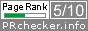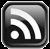# LEARN MS-ACCESS TIPS AND TRICKS

Learn Microsoft Access Advanced Programming Techniques, Tips and Tricks.

### Introduction.

If you have IBM iSeries Machine (IBM AS400) and taking raw data from there for customized report preparation in Microsoft Access then you might have noticed that the date value they provide will look like an ordinary number in mmddyyyy format (07092011) or in ddmmyyyy (09072011) or like yyyymmdd (20110709).  We cannot directly use this number as a date in a Query or anywhere else for analysis purposes. It should be converted into a real date before we can use them.

Let us try one or two conversion examples.

#### Example-1: mmddyyyy

x = 07092011 or x=”07092011”

y = July 09, 2011 (internal representation of the actual date number is 40733)

Conversion expression:

y = DateSerial(Right(x,4),Left(x,2),Mid(x,3,2))

OR

y = DateValue(Left(x,2) & "-" & Mid(x,3,2) & "-" & Right(x,4))

Depending on the input date format (Asian, USA or ANSI) usage order of Left(), Right() and Mid() functions in the DateSerial() or DateValue() Functions will change.  But, writing the above expressions every time when we put the data into Queries is not advisable and time consuming too.  The easiest way to overcome this is to create User-defined functions with the above expressions and call the function from the Query Column or from anywhere these date numbers are involved in calculations or comparisons etc.

#### The DMY_N2D() Function.

Let us create the following simple Functions that will make our life easier with these numbers in the long run.

Function: DMY_N2D(ByVal ddmmyyyy as Variant) As Date

Converts from date number in ddmmyyyy format into a real date number.  The input number can be in Long Number format (09072011 or 9072011) or in text format “09072011”.  _N2D stands for Number to Date.  The first three characters of the function name DMY indicates the order of Day, Month & Year elements of the input date number.

```Public Function DMY_N2D(ByVal ddmmyyyy As Variant) As Date
'------------------------------------------------------------------
'Converts Numbers (in ddmmyyyy format) 09072011 into a Date Number
'Author : a.p.r.pillai
'Date   : Sept. 1999
'------------------------------------------------------------------
Dim strN2D As String

On Error GoTo DMY_N2D_Err

strN2D = Format(ddmmyyyy, "00000000") ' add 0 at the left side, if 7 digit number

DMY_N2D = DateSerial(Right(strN2D, 4), Mid(strN2D, 3, 2), Left(strN2D, 2))

DMY_N2D_Exit:
Exit Function

DMY_N2D_Err:
MsgBox Err.Description,, "DMY_N2D()"
Resume DMY_N2D_Exit
End Function```

#### The MDY_N2D() Function.

When the input Number is in mmddyyyy format:

```Public Function MDY_N2D(ByVal mmddyyyy As Variant) As Date
'------------------------------------------------------------------
'Converts Numbers (in mmddyyyy format) 07092011 into a Date Number
'Author : a.p.r.pillai
'Date   : Sept. 1999
'------------------------------------------------------------------
Dim strN2D As String

On Error GoTo MDY_N2D_Err

strN2D = Format(mmddyyyy, "00000000") ' add 0 at the left side, if 7 digit number

MDY_N2D = DateSerial(Right(strN2D, 4), Left(strN2D, 2), Mid(strN2D, 3, 2))

MDY_N2D_Exit:
Exit Function

MDY_N2D_Err:
MsgBox Err.Description,, "MDY_N2D()"
Resume MDY_N2D_Exit
End Function```

#### The YMD_N2D() Function.

When the date number is in yyyymmdd (ANSI) format:

```Public Function YMD_N2D(ByVal yyyymmdd As Variant) As Date
'------------------------------------------------------------------
'Converts Numbers (in yyyymmdd format) 20110709 into a Date Number
'Author : a.p.r.pillai
'Date   : Sept. 1999
'------------------------------------------------------------------
Dim strN2D As String

On Error GoTo YMD_N2D_Err

YMD_N2D = DateSerial(Left(strN2D, 4),Mid(strN2D, 5, 2),Right(strN2D, 2))

DMY_N2D_Exit:
Exit Function

YMD_N2D_Err:
MsgBox Err.Description,, "YMD_N2D()"
Resume YMD_N2D_Exit
End Function```

Share:

Comments subject to moderation before publishing.

## TranslateSubscribe in a readerYour email address:

Delivered by FeedBurner